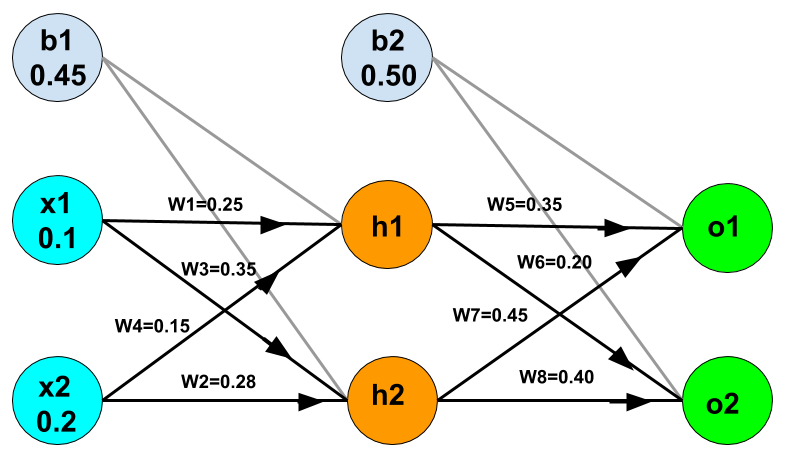### How Do Neural Networks Learn?

In this post, we focus on the neural network learning. How neural network learns. In a neural network we may have many output nodes. The cost function for neural network can be a generalized form of the cost function that we used in logistic regression. The difference is that here we have more nested summation for multiple output nodes. In the neural network the parameter matrix is very important, here we denote it with theta. The number of columns in parameter or theta matrix is equal to the number of nodes in the current layer including the bias unit and the number of rows is equal to the number of nodes in next layer excluding the bias unit.

In the previous post, we have given a gentle introduction of the Artificial Neural Network for Machine Learning. Make sure you have gone through the post and have learned the basics of the neural networks. The neural networks are gaining popularity in the Artificial Intelligence community.

## Backpropagation

Backpropagation is used for minimizing cost function. In backpropagation the error for a node in the current layer is calculated from the errors in the next layer. Mean the errors are propagated backward. These errors are denoted with delta so we call them delta values. These delta values are used to calculate partial derivatives of the cost function. In back propagation we calculate errors for all notes in all layers. The delta values (errors) of the layer l are calculated by multiplying the delta values in the next layer with theta matrix of layer l, then multiply element wise with derivative of activation function evaluated with the input given by the preceding layers.Fig: Backpropagation, Image Source.

The partial derivative terms can be calculated by multiplying activation values and the error values for each training example. The derivative is the slope of a line tangent to the cost function, so the steeper the slope the more incorrect values we will get. If we will get steeper slope we will go far from the slope.

To use optimizing functions we have to put all the theta parameters in one vector and the derivatives parameters in other vector. This we refer as unrolling the parameters.

Gradient checking is used to ensure that our backpropagation is working correctly or not. Derivatives obtained using the errors(delta) are put in a delta vector. In gradient checking we obtain the gradients (derivatives) from the approximation. If these two derivatives are same the it ensured that our backpropagation is going correct.

### Random Initialization

If we initialize all theta weights with zero then the backprpation will not work because when we backpropagate, all nodes will update to same value again and again. So we have to randomly initialize the weights.

### Network Architecture

To choose a network architecture or layout for neural network is most essential. How to choose how many layers, how many nodes in each layers? There is exact answer but the default is 1 hidden layer. But if choosing more hidden layers then the same number of nodes in every hidden layer.

### Training a Neural Network

Steps:

1. Randomly initialize the weights.

2. Implement forward propagation to get hypothesis value.

3. Implement the cost function.

4. Implement the backpropagation to compute the partial derivatives.

5. Use gradient checking to confirm that our backpropagation works properly. Then disable gradient checking.

6. Use gradient descent or any other optimization function to minimize the cost function with the weights.